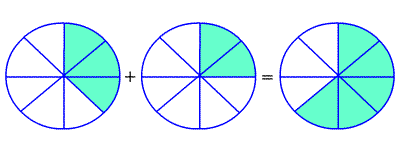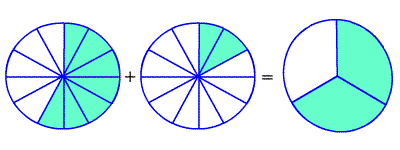# Adding Fractions with Like Denominators

Fractions with the same denominators are called like fractions .

To add fractions with like denominators, add the numerators , and write the sum over the denominator.

$\frac{3}{8}+\frac{2}{8}=\frac{5}{8}$Example :

Find $\frac{4}{9}+\frac{3}{9}$ .

Since the denominators are the same, add the numerators.

$\frac{4+3}{9}$

$=\frac{7}{9}$

You may get an answer which is not in lowest terms , even if the fractions you were adding and subtracting both were. In this case, you have to reduce the fraction .

$\frac{7}{12}+\frac{1}{12}=\frac{8}{12}=\frac{8\text{\hspace{0.17em}}÷\text{\hspace{0.17em}}4}{12\text{\hspace{0.17em}}÷\text{\hspace{0.17em}}4}=\frac{2}{3}$